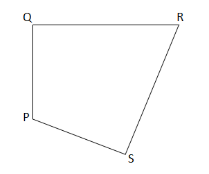QUESTION

# In a quadrilateral PQRS(i) Name the sides, angles, vertices and diagonals.(ii) Also name all the pairs of adjacent sides, adjacent angles, opposite sides and opposite angles.

Hint: In order to solve the problem use the figure and simple visualization. Basic definitions of quadrilateral will be used to solve the second part of the problem.

Sides: $PQ,QR,RS,SP$
Angles: $\angle PQR,\angle QRS,\angle RSP,\angle SPQ$
Vertices: $P,Q,R,S$
Diagonal: $PR,QS$

$\left\{ {PQ,QR} \right\},\left\{ {QR,RS} \right\},\left\{ {RS,SP} \right\},\left\{ {SP,PQ} \right\}$
$\left\{ {\angle PQR,\angle QRS} \right\},\left\{ {\angle QRS,\angle RSP} \right\},\left\{ {\angle RSP,\angle SPQ} \right\},\left\{ {\angle SPQ,\angle PQR} \right\}$
$PS,QR{\text{ and }}QP,RS$
$\angle QRS,\angle SPQ{\text{ and }}\angle RSP\angle PQR$